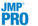Publication date: 07/30/2020

## Save Columns

Save Prediction Formula

For each Y variable, saves a column to the data table called Pred Formula <response> that contains the prediction formula.

Save Prediction as X Score Formula

For each Y variable, saves a column to the data table called Pred Formula <response> that contains the prediction formula in terms of the X scores.

Save Standard Errors of Prediction Formula

For each Y variable, saves a column to the data table called PredSE <response> that contains the standard error of the predicted mean. See Standard Error of Prediction and Confidence Limits.

Save Mean Confidence Limit Formula

For each Y variable, saves two columns to the data table called Lower 95% Mean <response> and Upper 95% Mean <response>. These columns contain 95% confidence limits for the response mean. See Standard Error of Prediction and Confidence Limits.

Save Indiv Confidence Limit Formula

For each Y variable, saves two columns to the data table called Lower 95% Indiv <response> and Upper 95% Indiv <response>. These columns contain 95% prediction limits for individual values. See Standard Error of Prediction and Confidence Limits.

Save Score Formula

Saves two sets of columns to the data table:

Columns called X Score <N> Formula containing the formulas for each X Score.

Columns called Y Score <N> Formula containing the formulas for each Y Score

Save Y Predicted Values

Saves the predicted values for the Y variables to columns in the data table.

Save Y Residuals

Saves the residual values for the Y variables to columns in the data table.

Save X Predicted Values

Saves the predicted values for the X variables to columns in the data table.

Save X Residuals

Saves the residual values for the X variables to columns in the data table.

Save Percent Variation Explained For X Effects

Saves the percent variation explained for each X variable across all extracted factors to a new data table.

Save Percent Variation Explained For Y Responses

Saves the percent variation explained for each Y variable across all extracted factors to a new data table.

Save Scores

Saves the X and Y scores for each extracted factor to the data table.

Save Standardized Scores

Saves the X and Y standardized scores used in constructing the Correlation Loading Plot to the data table. For the formulas, see Standardized Scores and Loadings.

Save T Square

Saves a column to the data table that contains the T2 formulas. The values in this column are also used in the T Square Plot.

Save Distance

Saves the Distance to X Model (DModX) and Distance to Y Model (DModY) values to the data table. These are the values used in the Distance Plots.

Save X Weights

Saves the weights for each X variable across all extracted factors to a new data table.Save Validation

Saves a new column to the data table describing how each observation was used in validation. For Holdback validation, the column identifies if a row was used for training or validation. For KFold validation, the column identifies the number of the subgroup to which the row was assigned.Save Imputation

If Impute Missing Data is selected, opens a new data table that contains the data table columns specified as X and Y, with missing values replaced by their imputed values. Columns for polynomial terms are not shown. If a Validation column is specified, the validation column is also included.Publish Prediction Formula

Creates prediction formulas and saves them as formula column scripts in the Formula Depot platform. If a Formula Depot report is not open, this option creates a Formula Depot report. See Formula Depot in Predictive and Specialized Modeling.Publish Score Formula

Creates X and Y score formulas and saves them as formula column scripts in the Formula Depot platform. If a Formula Depot report is not open, this option creates a Formula Depot report. See Formula Depot in Predictive and Specialized Modeling.